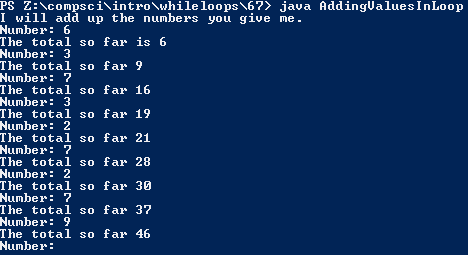# Assignment #67

## Code

```    /// Name: Kabir Virk
/// Period: 6
/// Program Name: Adding Values in a Loop
/// Date Finished: 12/16/2015

import java.util.Scanner;

{
public static void main(String[] args)
{
Scanner keyboard = new Scanner(System.in);

int number;
int total = 0;

System.out.println("I will add up the numbers you give me.");
System.out.print("Number: ");
number = keyboard.nextInt();

total = total + number;

if (number != 0)
System.out.println("The total so far is " + total);

while (number != 0)
{
System.out.print("Number: ");
number = keyboard.nextInt();

total = total + number;

if (number != 0)
System.out.println("The total so far " + total);
}

System.out.println();
System.out.println("The total is " + total);
}
}
```

### Picture of the output